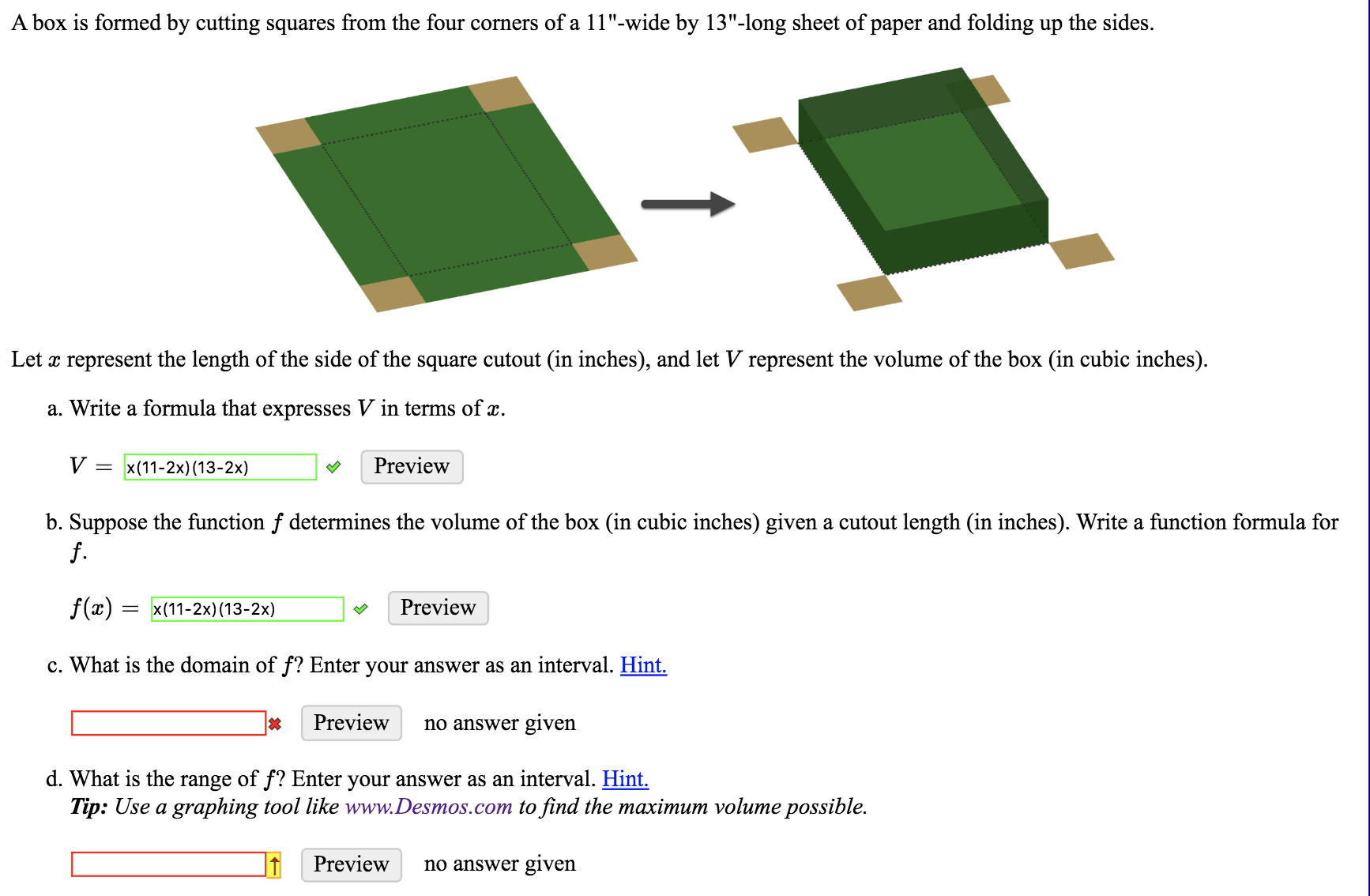# A box is formed by cutting squares from the four corners of a 11"-wide by 13"-long sheet of paper and folding up the sides.Let x represent the length of the side of the square cutout (in inches), and let V represent the volume of the box (in cubic inches)a. Write a formula that expresses V in terms of x.VPreviewx (11-2x) (13-2x)b. Suppose the function f determines the volume of the box (in cubic inches) given a cutout length (in inches). Write a function formula forf.f(x) = x(11-2x) (13-2x)Previewc. What is the domain of f? Enter your answer as an interval. Hint.Previewno answer givend. What is the range of f? Enter your answer as an interval. Hint.Tip: Use a graphing tool like www.Desmos.com to find the maximum volume possible.Previewno answer given

Question

Please respond to the boxes marked in red.help_outlineImage TranscriptioncloseA box is formed by cutting squares from the four corners of a 11"-wide by 13"-long sheet of paper and folding up the sides. Let x represent the length of the side of the square cutout (in inches), and let V represent the volume of the box (in cubic inches) a. Write a formula that expresses V in terms of x. V Preview x (11-2x) (13-2x) b. Suppose the function f determines the volume of the box (in cubic inches) given a cutout length (in inches). Write a function formula for f. f(x) = x(11-2x) (13-2x) Preview c. What is the domain of f? Enter your answer as an interval. Hint. Preview no answer given d. What is the range of f? Enter your answer as an interval. Hint. Tip: Use a graphing tool like www.Desmos.com to find the maximum volume possible. Preview no answer given fullscreen
check_circleExpert Solution
Step 1

Given, function is

Step 2

As, the function is the volume of box, which can’t be negative.

Step 3

Now, finding the doma...

### Want to see the full answer?

See Solution

#### Want to see this answer and more?

Solutions are written by subject experts who are available 24/7. Questions are typically answered within 1 hour*

See Solution
*Response times may vary by subject and question
Tagged in

### Other